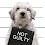## Wednesday, November 14, 2012

### Wednesday Brain Teaser - Driving down the highway

If the probability of observing a car in 30 minutes on a highway is 0.95, what is the probability of observing a car in 10 minutes (assuming constant default probability)?

Answer will be posted in the comment section sometime on Friday.

1.I don't know what constant default probability means. I also don't know that "observing a car" has any different meaning than "seeing a blue Mercedes."

Absent that, I don't see any verbal tricks and I don't see why it should be more complicated than a simple ratio.

2.If the highway is I-20 15 miles west of Pecos TX, the answer is zero.

No verbal tricks perhaps, but also not enough information unless that information is contained in the meaning of "constant default probability". If the smallest unit of time is less than 10 minutes, there are more than 3 "10 minutes" in 30 minutes. So first you'd have to know the probability of seeing a car within the first smallest unit of the 30 because that's the only time that isn't included in one or more of the rest of the 10 minute segments.

If the smallest unit of time is 10 minutes, it's a different problem, isn't it?

And then there's the probability that I have no idea what I'm talking about because this is maths, right?

3.Constant default probability - the probability at all time points is equal

Location - not Texas! Or New England apparently....I live right next to 2 of our biggest highways and the better question would be "what's the chance you're completely at a standstill because a minor fender bender has backed traffic up for 6 miles while everyone stares?"

4.If you'd like to get really technical, the arrival of cars in this problem follows a Poisson distribution:
http://en.wikipedia.org/wiki/Poisson_distribution

5.If it's a Poisson distibution, it's going to come out around 40% rather than 31.7, I'm thinking. Too lazy to do it out.

6.The first trick is to realize you have to come at it from the angle of the probability of not seeing a car, as the .95 is for seeing any number of cars and thus can not be extrapolated from. The probability of not seeing a car will then be 1 - probability of seeing a car.

If P(a) = the probability of not seeing a car in 30 minutes
P(b) = the probability of not seeing a car in 10 minutes

Then we first convert the given probability of seeing a car in to the probability of not seeing a car:
P(a) = 1 - .95 = .05

Since we know that the probability is equal at all time points, and since there are 3 10 minute segments in 30 minutes we get
P(b) * P(b) * P(b) = P(a)

or

P(b)^3 = P(a)

Taking the above we get

P(b)^3 = .05

So P(b) = cube root of .05 = .368

Thus 1- P(b) = 1 - .368 = about .63

So in any given 10 minutes, you have a 63% chance of seeing a car.

7.Completely different: On T-day, I drove up to Seattle. Was passed by a car with license plate O-HUSH. Returning Sunday, same car passed us.